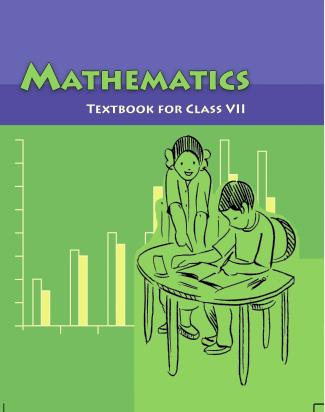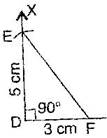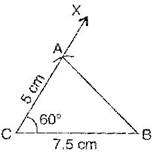1. /
2. CBSE
3. /
4. Class 07
5. /
6. Mathematics
7. /
8. NCERT Solutions for Class...

# NCERT Solutions for Class 7 Maths Exercise 10.3### myCBSEguide App

Download the app to get CBSE Sample Papers 2023-24, NCERT Solutions (Revised), Most Important Questions, Previous Year Question Bank, Mock Tests, and Detailed Notes.

NCERT solutions for Maths Practical Geometry## NCERT Solutions for Class 7 Maths Practical Geometry

###### Question 1.Construct {tex}\Delta {/tex}DEF such that DE = 5 cm, DF = 3 cm and {tex}m\angle {/tex}EDF = {tex}{90^ \circ }.{/tex}

To construct: {tex}\Delta {/tex}DEF where DE = 5 cm, DF = 3 cm and {tex}m\angle {/tex}EDF = {tex}{90^ \circ }.{/tex}Steps of construction:

(a) Draw a line segment DF = 3 cm.

(b) At point D, draw an angle of {tex}{90^ \circ }{/tex} with the help of compass i.e., {tex}\angle {/tex}XDF = {tex}{90^ \circ }{/tex}.

(c) Taking D as centre, draw an arc of radius 5 cm, which cuts DX at the point E.

(d) Join EF.

It is the required right angled triangle DEF.

NCERT Solutions for Class 7 Maths Exercise 10.3

###### Question 2.Construct an isosceles triangle in which the lengths of each of its equal sides is 6.5 cm and the angle between them is {tex}110^\circ .{/tex}

To construct: An isosceles triangle PQR where PQ = RQ = 6.5 cm and {tex}\angle {/tex}Q = {tex}110^\circ .{/tex}

Steps of construction:

(a) Draw a line segment QR = 6.5 cm.

(b) At point Q, draw an angle of {tex}110^\circ {/tex} with the

help of protractor, i.e., {tex}\angle {/tex}YQR = {tex}110^\circ .{/tex}

(d) Taking Q as centre, draw an arc with radius

1. cm, which cuts QY at point P. 110{tex}^\circ {/tex}

(e) Join PR

It is the required isosceles triangle PQR.

NCERT Solutions for Class 7 Maths Exercise 10.3

###### Question 3.Construct {tex}\Delta {/tex}ABC with BC = 7.5 cm, AC = 5 cm and {tex}m\angle {/tex}C = {tex}{60^ \circ }.{/tex}

To construct: {tex}\Delta {/tex}ABC where BC = 7.5 cm, AC = 5 cm and {tex}m\angle {/tex}C = {tex}{60^ \circ }.{/tex}###### Steps of construction:

(a) Draw a line segment BC = 7.5 cm.

(b) At point C, draw an angle of {tex}{60^ \circ }{/tex} with the help of protractor, i.e., {tex}\angle {/tex}XCB = {tex}{60^ \circ }.{/tex}

(c) Taking C as centre and radius 5 cm, draw an arc, which cuts XC at the point A.

(d) Join AB

It is the required triangle ABC.

## NCERT Solutions for Class 7 Maths Exercise 10.3

NCERT Solutions Class 7 Maths PDF (Download) Free from myCBSEguide app and myCBSEguide website. Ncert solution class 7 Maths includes text book solutions from Class 7 Maths Book . NCERT Solutions for CBSE Class 7 Maths have total 15 chapters. 7 Maths NCERT Solutions in PDF for free Download on our website. Ncert Maths class 7 solutions PDF and Maths ncert class 7 PDF solutions with latest modifications and as per the latest CBSE syllabus are only available in myCBSEguide.

## CBSE app for Students

To download NCERT Solutions for Class 7 Maths, Social Science Computer Science, Home Science, Hindi English, Maths Science do check myCBSEguide app or website. myCBSEguide provides sample papers with solution, test papers for chapter-wise practice, NCERT solutions, NCERT Exemplar solutions, quick revision notes for ready reference, CBSE guess papers and CBSE important question papers. Sample Paper all are made available through the best app for CBSE students and myCBSEguide website.Test Generator

Create question paper PDF and online tests with your own name & logo in minutes.myCBSEguide

Question Bank, Mock Tests, Exam Papers, NCERT Solutions, Sample Papers, Notes

### 1 thought on “NCERT Solutions for Class 7 Maths Exercise 10.3”

1. Best guide in the syllbus
???????????